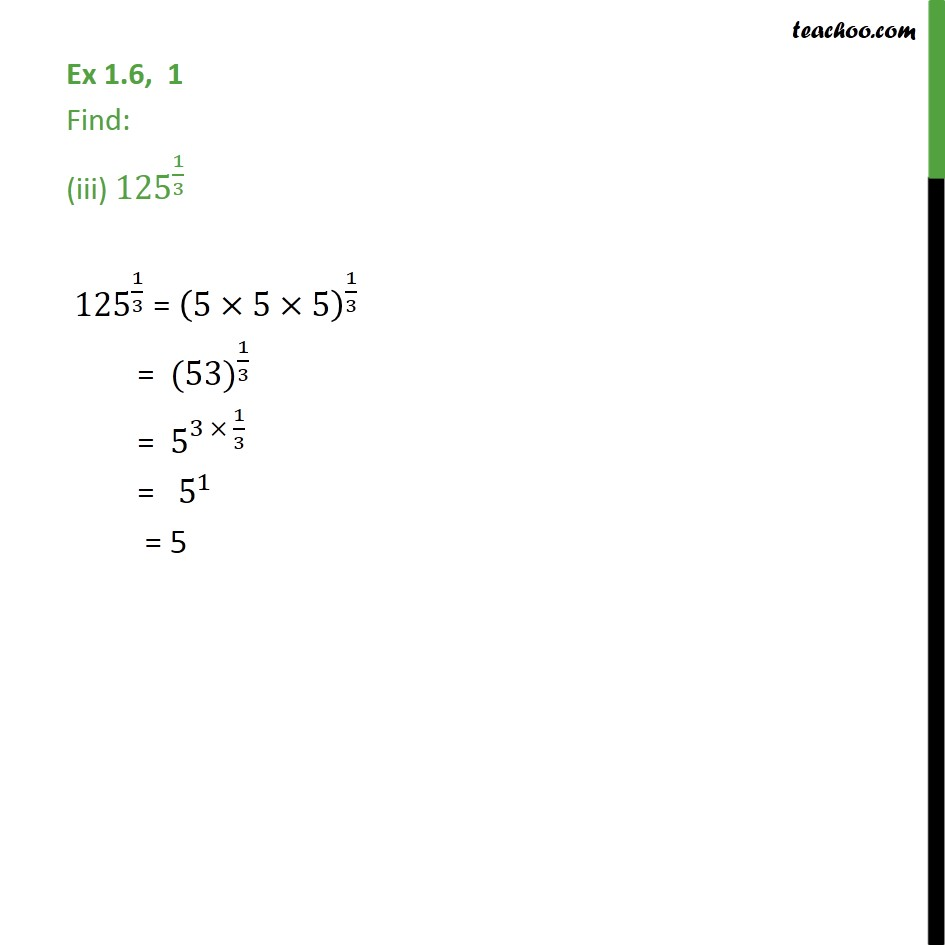1. Chapter 1 Class 9 Number Systems (Term 1)
2. Serial order wise
3. Ex 1.6

Transcript

Ex 1.6, 1 Find: (iii) 〖125〗^(1/3) 〖 125〗^(1/3) = (5×5×5)^(1/3) = 〖 (53)〗^(1/3) = 〖 5〗^(3 × 1/3) = " " 〖 5〗^(1 ) = 5